## Reasoning Practice Test 1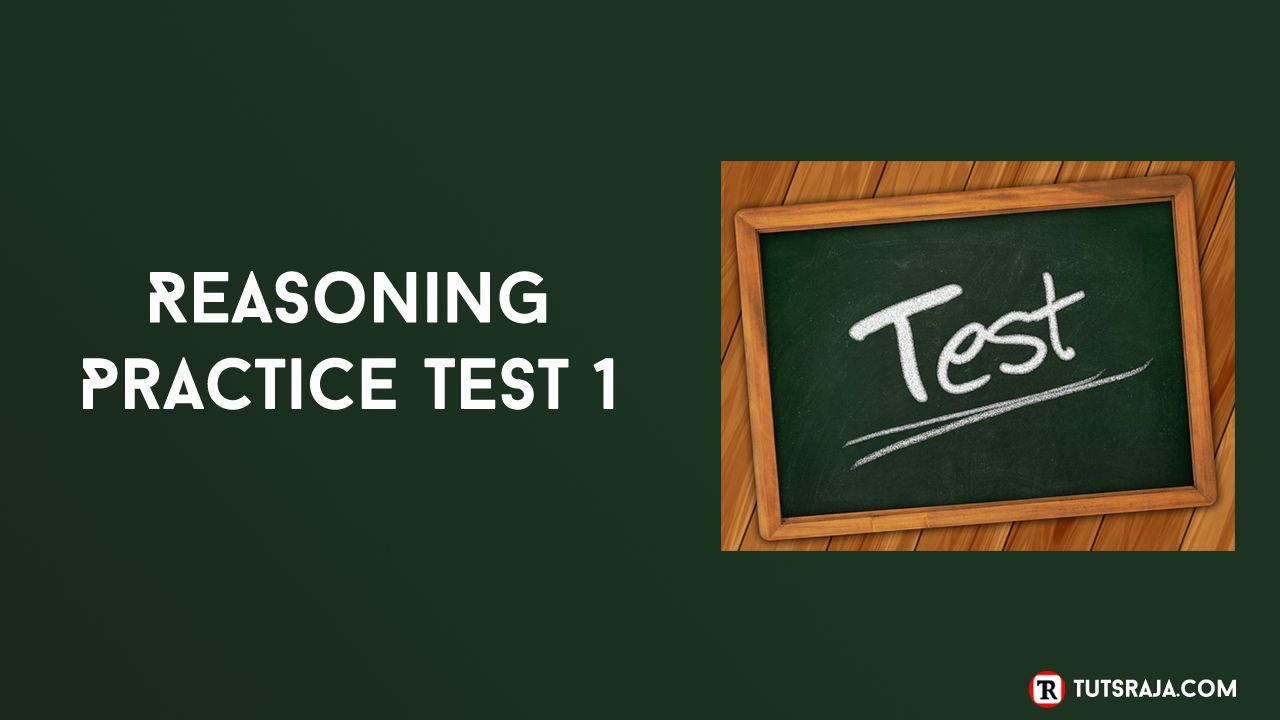Reasoning Practice Test is designed to test your knowledge and accuracy. Here is a daily free online practice test with question and answers. Finally, test your knowledge and improve your scores.

All the best for the practice test. Do you know How remember letter positions with tricks ?

Let's Start,

1. In a certain code language, DANGER is written as FCPIGT, then how HORRIBLE written in same code language ?

Solution:Here, Each letter of word is moved two steps forward to obtain the result letter of the code.

2. If WINDOW is coded as DRMWLD and ROOF is coded as ILLU. How HOUSE written in that code ?

Solution: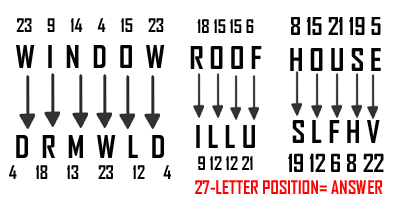Here, Result letter of word is reverse order. Use formula 27-(letter postion)=answer to obtain the result letter of the code.

3. If GREEN is coded as GSEFN, How is ORANGE coded in that code ?

Solution:Here, odd letter position is same and even letter position is moved one step forword.

4. If YELLOW is coded as JCWVMJ and BRIGHT is coded as GPZRFE. How MONKEY written in that code ?

Solution:Here, position of word letter is moved two steps backward. Structure of backward position is two digit shortcut multiplication.

5. In a certain code language MONEY is written as BDCEC. How will POWER be written in that code language ?

Solution: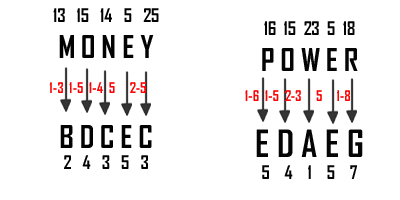Here, result position of word letter is substraction of individual digits.

6. In a certain code language ADDRESS is written as HWWIHVZ. How will ANYBODY be written in that code language ?

Solution: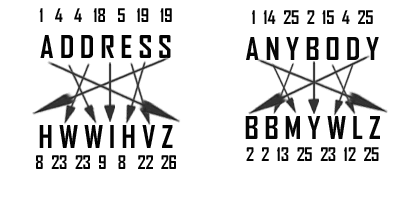Here, result position of word letter is reverse order. Structure of letters is two digit numerical multiplication.

7. In a certain code language PLANS is written as RMZKO. How will PAPER be written in that code language ?

Solution:Here, result position of word letter is moved one step backward. Structure of letters is star.

8. In a certain code language RANGER is written as DUIPSF. How will PEOPLE be written in that code language ?

Solution:Here, result position of word letter is moved (+3 +3 +2 +2 +1 +1) step forward. Structure of letters is cross .

9. In a certain code language, ADVISER is written as BFYMXKY, then how ANOTHER written in same code language ?

Solution:Here, result position of word letter is moved (+1 +2 +3 +4 +5 +6 +7) step forward.

10. In a certain code language, ACCOUNT is written as DEDOVPW, then how AIRPORT written in same code language ?

Solution: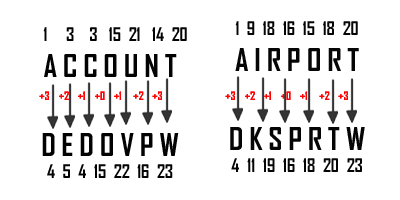Here, result position of word letter is moved (+3 +2 +1 0 +1 +2 +3) step forward. Structure of letters is integer numbers .RajashekarKankanala
Tuts Raja
NTR Colony
9110760272
http://www.tutsraja.com/

Share post»